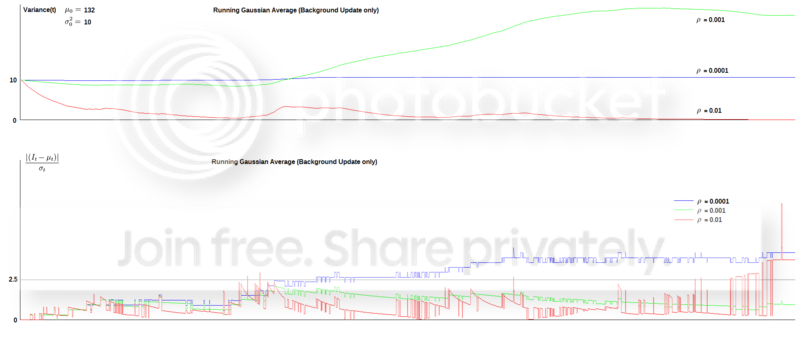# How to select a good window size in the Running Gaussian Average method for Selective Background Updating?

I have been studying the Selective Background Updating in Running Gaussian Average Method, as discussed in the Wikipedia (the Variant method). The details of the algorithm, as I understand, is as follows:

For each pixel, at initialization $(t=0)$, set $\mu_0=I_0$ (the initial intensity of the pixel), and $\sigma^2_t=c$ (here I use $c = 10$).

At each turn $t$, calculate the following in order for each pixel:

$\mu_t=\rho I_t + (1-\rho) \mu_{t-1}$

$\sigma^2_t=(I_t - \mu_t)^2 \rho + (1-\rho) \sigma^2_{t-1}$

$q_t = |I_t - \mu_t| / \sigma_t$

If $q_t \gt k \rightarrow$ pixel is foreground, and we don't update the mean and variance

(i.e. $\mu_t=\mu_{t-1}$ and $\sigma^2_t=\sigma^2_{t-1}$),

else pixel is background.

I have written a small C program to test the effect of different $\rho$ values. The input data is a 1-D array of nearly 2000 elements, with values ranging from 132 to 147, with a generally gradually increasing trend (but sometimes have an occasion drop of 1 or 2), to represent a slightly increasing light intensity on the background. Three different values of $\rho$: 0.0001, 0.001 and 0.01 are used, and for each of the three cases, two graphs are then plotted: Variance $\sigma^2_t$ against $t$ (above), and $q_t$ against $t$ (below). The plots look like this (click link to see the enlarge version):To my surprise, when $\rho = 0.01$ (a short history), the variance becomes so small $(<0.2)$ near the end, that even when the next data increases or decreases by $1$, the value $|I_t - \mu_t| / \sigma_t$ becomes larger than $2.5$ and is hence considered a foreground. But when $\rho = 0.0001$ (a long history), the variance does not change much from the initial value $(10)$ at all. However, the best result in my case is when $\rho = 0.001$, where the variance becomes quite large $(\simeq25)$ at the end, making the value $|I_t - \mu_t| / \sigma_t$ always smaller than $2.5$, and is considered background correctly.

So it seems to me that while getting the correct $\rho$ is important, it is also a bit by luck. Would you tell me what is the criteria of getting a good $\rho$, or have I made any mistake in my program code?

• Please get rid of the source code and replace it with ideas, pseudo code and arguments of correctness. See here and here for related meta discussions. – Raphael Sep 18 '15 at 17:52
• You seem to get really into selective Gaussian, but to answer your question there are still things to consider: do you garantee gaussianity of intensity distribution? Did you got rid of luminance? Where data is comming from? What are conditions? Besides the luck there is also size of object you should consider. Why this method? Could you do several separate windows for different times and shapes? Because if you put camera to look outside the window (from home) environment is noisy. If you have syntetic video you have proof that this method is hard to tune. – Evil Sep 20 '15 at 15:09
• @EvilJS I used selective Gaussian instead of a mixture of Gaussian method mainly because the background is not multi-modal. Moreover, I can actually have certain control on the initial situation, by making sure that no foreground object exists. However, my video frames are grayscale so I don't have three separate channels to work on. – GreenPenguin Sep 22 '15 at 15:07
• So unimodal model, syntetic game, initial background only - this simplifies much and also it is worth mentioning in your question. Constant illumination and lack of noise with tracking of constant number of objects. Well, to me it sound like perfect scheme to tracking moving objects, with really no need for accumulators on the whole scene. Maybe I misunderstood but it is more like tracking objects on constant background and adding new places to be background when you move? – Evil Sep 22 '15 at 16:07